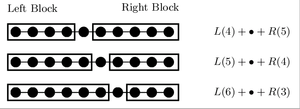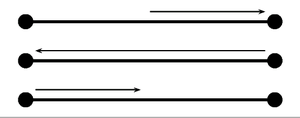# Density matrix renormalization group

Density matrix renormalization group

The density matrix renormalization group (DMRG) is a numerical variational technique devised to obtain the low energy physics of quantum many-body systems with high accuracy. It was invented in 1992 by Steven R. White and it is nowadays the most efficient method for 1-dimensional systems.

## The idea behind DMRG

The main problem of quantum many-body physics is the fact that the Hilbert space grows exponentially with size. For example, a spin-1/2 chain of length L has 2 L degrees of freedom. The DMRG is an iterative, variational method that reduces effective degrees of freedom to those most important for a target state. The target state is often the ground state.

After a warmup cycle, the method splits the system into two blocks, which need not have equal sizes, and two sites in between. A set of representative states has been chosen for the block during the warmup. This set of left block + two sites + right block is known as the superblock. Now a candidate for the ground state of the superblock, which is a reduced version of the full system, may be found. It may have a rather poor accuracy, but the method is iterative and improves with the steps below.Decomposition of the system into left and right blocks, according to DMRG.

The candidate ground state that has been found is projected into the subspace for each block using a density matrix, whence the name. Thus, the relevant states for each block are updated.

Now one of the blocks grows at the expense of the other and the procedure is repeated. When the growing block reaches maximum size, the other starts to grow in its place. Each time we return to the original (equal sizes) situation, we say that a sweep has been completed. Normally, a few sweeps are enough to get a precision of a part in 1010 for a 1D lattice.The DMRG sweep.

The first application of the DMRG, by Steven White and Reinhard Noack, was a toy model: to find the spectrum of a spin 0 particle in a 1D box. This model had been proposed by Kenneth G. Wilson as a test for any new renormalization group method, because they all happened to fail with this simple problem. The DMRG overcame the problems of previous renormalization group methods by connecting two blocks with the two sites in the middle rather than just adding a single site to a block at each step as well as by using the density matrix to identify the most important states to be kept at the end of each step. After succeeding with the toy model, the DMRG method was tried with success on the Heisenberg model (quantum).

## Technical details about the implementation

A practical implementation of the DMRG algorithm is a lengthy work. A few of the main computational tricks are these:

• The ground state for the superblock is obtained using the Lanczos algorithm of matrix diagonalization. Another choice is the Arnoldi method, especially when dealing with non-hermitian matrices.
• The Lanczos algorithm usually starts with a random seed. In DMRG, the ground state obtained in a certain DMRG step, suitably transformed, may serve as a better seed for the Lanczos algorithm at the next DMRG step.
• In systems with symmetries, we may have conserved quantum numbers, such as total spin in a Heisenberg model (quantum). It is convenient to find the ground state within each of the sectors into which the Hilbert space is divided.

## Applications

The DMRG has been successfully applied to get the low energy properties of spin chains: Ising model in a transverse field, Heisenberg model, etc., fermionic systems, such as the Hubbard model, problems with impurities such as the Kondo effect, boson systems, and the physics of quantum dots joined with quantum wires. It has been also extended to work on tree graphs, and has found applications in the study of dendrimers. For 2D systems with one of the dimensions much larger than the other DMRG is also accurate, and has proved useful in the study of ladders.

The method has been extended to study equilibrium statistical physics in 2D, and to analyze non-equilibrium phenomena in 1D.

The DMRG has also been applied to the field of Quantum Chemistry to study strongly correlated systems.

## The matrix product ansatz

The success of the DMRG for 1D systems is related to the fact that it is a variational method within the space of matrix product states. These are states of the form$\sum_{s_1\cdots s_N} \operatorname{Tr}(A^{s_1}\cdots A^{s_N}) | s_1 \cdots s_N\rangle$

where$s_1\cdots s_N$ are the values of the, e.g. z-component of the spin in a spin chain, and the Asi are matrices of arbitrary dimension m. As m → ∞, the representation becomes exact. This theory was exposed by S. Rommer and S. Ostlund in .

## Extensions of DMRG

In 2004 the time-evolving block decimation method was developed to implement real time evolution of Matrix Product States. The idea is based on the classical simulation of a quantum computer. Subsequently, a new method was devised to compute real time evolution within the DMRG formalism - See the paper by A. Feiguin and S.R. White .

In recent years, some proposals to extend the method to 2D and 3D have been put forward, extending the definition of the Matrix Product States. See this paper by F. Verstraete and I. Cirac, .

• The original paper, by S. R. White,  or 
• A broad review, by Karen Hallberg, .
• Two reviews by Ulrich Schollwöck, one discussing the original formulation , and another in terms of matrix product states 
• The Ph.D. thesis of Javier Rodríguez Laguna .
• An introduction to DMRG and its time-dependent extension .
• A list of DMRG e-prints on arxiv.org .

## Related software

• Powder with Power: a free distribution of time-dependent DMRG code written in Fortran 
• The ALPS Project: a free distribution of time-independent DMRG code and Quantum Monte Carlo codes written in C++ 

Wikimedia Foundation. 2010.

### Look at other dictionaries:

• Renormalization group — In theoretical physics, the renormalization group (RG) refers to a mathematical apparatus that allows systematic investigation of the changes of a physical system as viewed at different distance scales. In particle physics, it reflects the… …   Wikipedia

• Renormierungsgruppe — Die Renormierungsgruppe (RG) beschreibt die Abhängigkeit bestimmter physikalischer Größen von der Energieskala. Ursprünglich ein Konzept der Quantenfeldtheorie, erstreckt sich sein Anwendungsbereich heutzutage auch auf die Festkörperphysik,… …   Deutsch Wikipedia

• Dmrg of Heisenberg model — This example presents the infinite DMRG algorithm. It is about S = 1 antiferromagnetic Heisenberg chain, but the recipe can be applied for every translationally invariant one dimensional lattice. DMRG is a renormalization group technique because… …   Wikipedia

• Time-evolving block decimation — The time evolving block decimation (TEBD) algorithm is a numerical scheme used to simulate one dimensional quantum many body systems, characterized by at most nearest neighbour interactions.It is dubbed Time evolving Block Decimation because it… …   Wikipedia

• Steven R. White — (born December 26, 1959 in Lawton, Oklahoma) is the professor of physics at the University of California at Irvine. He graduated from University of California at San Diego; he then received his Ph.D. at Cornell University. He is most known for… …   Wikipedia

• Quantum Monte Carlo — is a large class of computer algorithms that simulate quantum systems with the idea of solving the many body problem. They use, in one way or another, the Monte Carlo method to handle the many dimensional integrals that arise. Quantum Monte Carlo …   Wikipedia

• Witold Nazarewicz — (* 26. Dezember 1954 in Warschau), ist ein polnischer Kernphysiker. Inhaltsverzeichnis 1 Leben 2 Schriften 3 Weblinks …   Deutsch Wikipedia

• Ising model — The Ising model, named after the physicist Ernst Ising, is a mathematical model in statistical mechanics. It has since been used to model diverse phenomena in which bits of information, interacting in pairs, produce collectiveeffects.Definition… …   Wikipedia

• Édouard Brézin — (b. December 1, 1938 Paris) is a French theoretical physicist. He is professor at Université Paris 6, working at the laboratory for theoretical physics (LPT) of the École normale supérieure.Édouard Brézin studied at École polytechnique before… …   Wikipedia

• List of mathematics articles (R) — NOTOC R R. A. Fisher Lectureship Rabdology Rabin automaton Rabin signature algorithm Rabinovich Fabrikant equations Rabinowitsch trick Racah polynomials Racah W coefficient Racetrack (game) Racks and quandles Radar chart Rademacher complexity… …   Wikipedia